Michel Albert

# A Lesson in Unit Testing

Thu 04 September 2014

Filed under programming

Quite some time back, I wrote a Python library for simple clustering tasks. Back in the day, I only needed K-Means clustering. But I decided I would add hierarchical clustering as well as it could come in handy later on.

Turns out I messed up the unit tests, and the hierarchical clustering algorithm. But the error went unnoticed as the unit-tests were not testing one obvious fact:

Are all the elements from the input present in the output? And only those from the input?

The main cause of this error is because the way I obtained the expected values was complete heresy! I ran the test run on random values, and simply copy/pasted the results into the expected values. Of course now the test passed, with the important fact that the tests were testing against the wrong values!

In order to fix address this, I first need some proper test values. So now I do what I should have done in the first place: Manually step through the algorithm. This article contains my scribbles which I will use as a "mental strut".

## Hierarchical Clustering

We will start of with a sample of non primitive data types. In this case points on a two-dimensional plane. As distance function we will use the euclidian distance. And, for the first step through, we will use single linkage between clusters. The same scenario will be played through with complete- and uclus-linkage later on. As overall method, we will use a bottom-up approach.

The three aproaches will result in the following dendograms: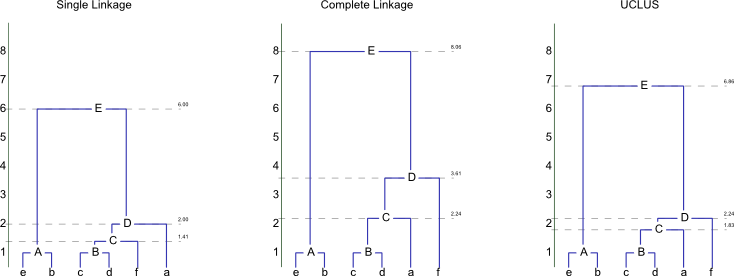### Initial Step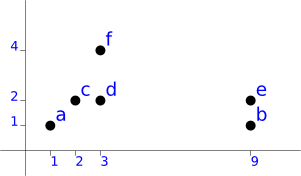So we have the following points:

```a (1, 1)
b (9, 1)
c (2, 2)
d (3, 2)
e (9, 2)
f (3, 4)
```

Note

To keep the matrix table more readable, individual points are written in lower-case, while clusters are written in upper-case!

This results in the following initial distance matrix. We will mark the diagonal with an x as we're not interested in comparing a point with itself. Equally, we are only interested in the absolute distance. So we only need the values for pairs on ons side of the diagonal.

Distance Matrix
a b c d e f
a x 8.00 1.41 2.24 8.06 3.61
b   x 7.07 6.08 1.00 6.71
c     x 1.00 7.00 2.24
d       x 6.00 2.00
e         x 6.32
f           x

#### First Step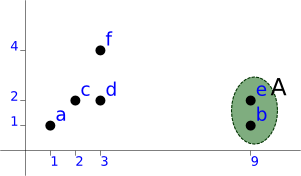With this matrix, we see that the first candidates are [b, e] and [c, d]. We'll pick [b, e] as firt cluster (A):

```a (1, 1)
A (9, 1), (9, 2) level=1.00
c (2, 2)
d (3, 2)
f (3, 4)
```
Distance Matrix
a A c d f
a x 8.00 1.41 2.24 3.61
A   x 7.00 6.00 6.32
c     x 1.00 2.24
d       x 2.00
f         x

#### Second Step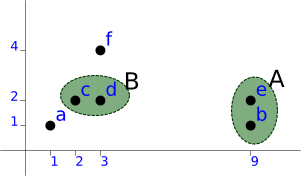The next candidate is [c, d] as B:

```a (1, 1)
A (9, 1), (9, 2) level=1.00
B (2, 2), (3, 2) level=1.00
f (3, 4)
```

Giving us:

Distance Matrix
a A B f
a x 8.00 1.41 3.61
A   x 6.00 6.32
B     x 2.00
f       x

#### Third Step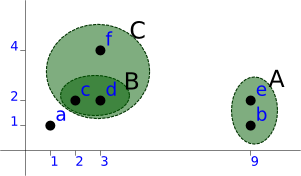Then [f, B] as C:

```a (1, 1)
A (9, 1), (9, 2) level=1.00
C (3, 4), ((2, 2), (3, 2) level=1.00) level=2.00
```

Resulting in:

Distance Matrix
a A C
a x 8.00 1.41
A   x 6.00
C     x

#### Fourth Step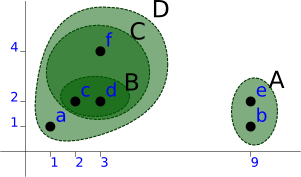Then [a, C] as D:

```A (9, 1), (9, 2) level=1.00
D (1, 1), ((3, 4), ((2, 2), (3, 2) level=1.00) level=2.00) level=1.41
```

Resulting in:

Distance Matrix
A D
A x 6.00
D   x

Which gives us the final cluster E with a level of 6.00.

The end-result is the following dendogram:

```               E
|
+-----------+-----------+
|                       |
|                       D
|                       |
|                 +-----+-----+
|                 |           |
|                 C           |
|                 |           |
|            +----+----+      |
|            |         |      |
A            B         |      |
|            |         |      |
+--+--+      +--+--+      |      |
|     |      |     |      |      |
e     b      c     d      f      a
```

Initial distance matrix for reference:

Distance Matrix
a b c d e f
a x 8.00 1.41 2.24 8.06 3.61
b   x 7.07 6.08 1.00 6.71
c     x 1.00 7.00 2.24
d       x 6.00 2.00
e         x 6.32
f           x

#### First Step

First iteration is identical, but distance matrix has different values. The subsequent steps will be displayed without aditional explanation, the idea is the same as above, simply using a different linkage function.Distance Matrix
a A c d f
a x 8.06 1.41 2.24 3.61
A   x 7.07 6.08 6.71
c     x 1.00 2.24
d       x 2.00
f         x

#### Second StepDistance Matrix
a A B f
a x 8.06 2.24 3.61
A   x 7.07 6.71
B     x 2.24
f       x

#### Third Step

Note

We now have to make a choice. I have not yet decided on how to handle this situation to have a detereministic behaviour. My current train of thought is using python sets as data-structure which is unordered. So the algorithm could return either one here.

For a demonstration, we'll pick [Ba] as to have a different result from sinle linkage...This will give us:

Distance Matrix
C A f
C x 8.06 3.61
A   x 6.71
f     x

#### Fourth StepAnd finally

Distance Matrix
D A
D x 8.06
A   x

The end-result is the following dendogram:

```               E
|
+-----------+-----------+
|                       |
|                       D
|                       |
|                 +-----+-----+
|                 |           |
|                 C           |
|                 |           |
|            +----+----+      |
|            |         |      |
A            B         |      |
|            |         |      |
+--+--+      +--+--+      |      |
|     |      |     |      |      |
e     b      c     d      a      f
```

### Uclus

Initial distance matrix for reference:

Distance Matrix
a b c d e f
a x 8.00 1.41 2.24 8.06 3.61
b   x 7.07 6.08 1.00 6.71
c     x 1.00 7.00 2.24
d       x 6.00 2.00
e         x 6.32
f           x

As in complete linkage, the first iteration is identical, but distance matrix has different values. So we will not go into too much detail and will skip the visualisations.

Distance Matrix
a A c d f
a x 8.03 1.41 2.24 3.61
A   x 7.04 6.04 6.52
c     x 1.00 2.24
d       x 2.00
f         x
Distance Matrix
a A B f
a x 8.03 1.83 3.61
A   x 6.54 6.52
B     x 2.12
f       x
Distance Matrix
C A f
C x 7.04 2.24
A   x 6.52
f     x

And finally

Distance Matrix
D A
D x 6.86
A   x

The end-result is the following dendogram:

```               E
|
+-----------+-----------+
|                       |
|                       D
|                       |
|                 +-----+-----+
|                 |           |
|                 C           |
|                 |           |
|            +----+----+      |
|            |         |      |
A            B         |      |
|            |         |      |
+--+--+      +--+--+      |      |
|     |      |     |      |      |
e     b      c     d      a      f
```